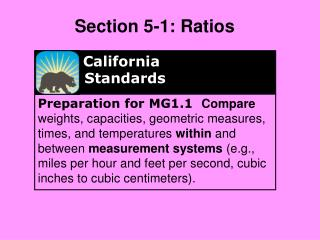DownloadDownload PresentationCalifornia Standards

# California Standards

Télécharger la présentation## California Standards

- - - - - - - - - - - - - - - - - - - - - - - - - - - E N D - - - - - - - - - - - - - - - - - - - - - - - - - - -
##### Presentation Transcript

1. California Standards Preparation for MG1.1 Compare weights, capacities, geometric measures, times, and temperatures within and between measurement systems (e.g., miles per hour and feet per second, cubic inches to cubic centimeters). Section 5-1: Ratios

2. Ratio Movie

3. 7 5 Notes A ratio is a comparison of two quantities. Ratios can be written in several ways. 7 to 5, 7:5, and name the same ratio. 12 inches = 1 foot 3 feet = 1 yard 36 inches = 1 yard

4. 15 ÷ 3 9 ÷ 3 bikes skateboards Additional Example 1: Writing Ratios in Simplest Form Write the ratio 15 bikes to 9 skateboards in simplest form. 15 9 Write the ratio as a fraction. = 5 3 Simplify. = = 5 3 The ratio of bikes to skateboards is , 5:3, or 5 to 3.

5. shirts jeans 24 ÷ 3 9 ÷ 3 Check It Out! Example 1 Write the ratio 24 shirts to 9 jeans in simplest form. Write the ratio as a fraction. 24 9 = 8 3 Simplify. = = 8 3 The ratio of shirts to jeans is , 8:3, or 8 to 3.

6. Practice • 15 cows to 25 sheep • 24 cars to 18 trucks • 30 Knives to 27 spoons

7. When simplifying ratios based on measurements, write the quantities with the same units, if possible.

8. 3 yards 12 feet 9 ÷ 3 12 ÷ 3 = = = 3 4 3 4 The ratio is , 3:4, or 3 to 4. Additional Example 2: Writing Ratios Based on Measurement Write the ratio 3 yards to 12 feet in simplest form. First convert yards to feet. 3 yards = 3 ● 3 feet There are 3 feet in each yard. Multiply. = 9 feet Now write the ratio. 9 feet 12 feet Simplify.

9. 36 inches 4 feet 36 ÷ 12 48 ÷ 12 = = = 3 4 3 4 The ratio is , 3:4, or 3 to 4. Check It Out! Example 2 Write the ratio 36 inches to 4 feet in simplest form. First convert feet to inches. 4 feet = 4 ● 12 inches There are 12 inches in each foot. = 48 inches Multiply. Now write the ratio. 36 inches 48 inches Simplify.

10. Practice • 4 feet to 24 inches • 3 yards to 12 feet • 2 yards to 20 inches

11. Notes Ratios that make the same comparison are equivalent ratios. Equivalent ratios represent the same point on the number line. To check whether two ratios are equivalent, you can write both in simplest form.

12. 1 9 1 9 12 15 3 27 27 36 2 18 Since , the ratios are equivalent. B. A. = and and 2 18 3 27 2 ÷ 2 18 ÷ 2 3 ÷ 3 27 ÷ 3 = = = = 4 5 3 4 Since , the ratios are not equivalent.  12 15 27 36 12 ÷ 3 15 ÷ 3 27 ÷ 9 36 ÷ 9 = = = = Additional Example 3: Determining Whether Two Ratios Are Equivalent Simplify to tell whether the ratios are equivalent. 1 9 1 9 4 5 3 4

13. Practice

14. 8 30 30 7 1 2 12 45 Possible answer: , Possible answer: , 4 15 7 21 3. 4. 1 3 14 42 Lesson Quiz: Part I Write each ratio in simplest form. 1. 22 tigers to 44 lions 2. 5 feet to 14 inches Find a ratios that is equivalent to each given ratio.

15. 36 24 16 10 5. 6. 8 64 16 128 and ; yes, both equal 28 18 32 20 8 5 3 2 14 9 8 5 1 8 =  ; yes ; no Lesson Quiz: Part II Simplify to tell whether the ratios are equivalent. and and 7. Kate poured 8 oz of juice from a 64 oz bottle. Brian poured 16 oz of juice from a 128 oz bottle. Are the ratios of poured juice to starting amount of juice equivalent?